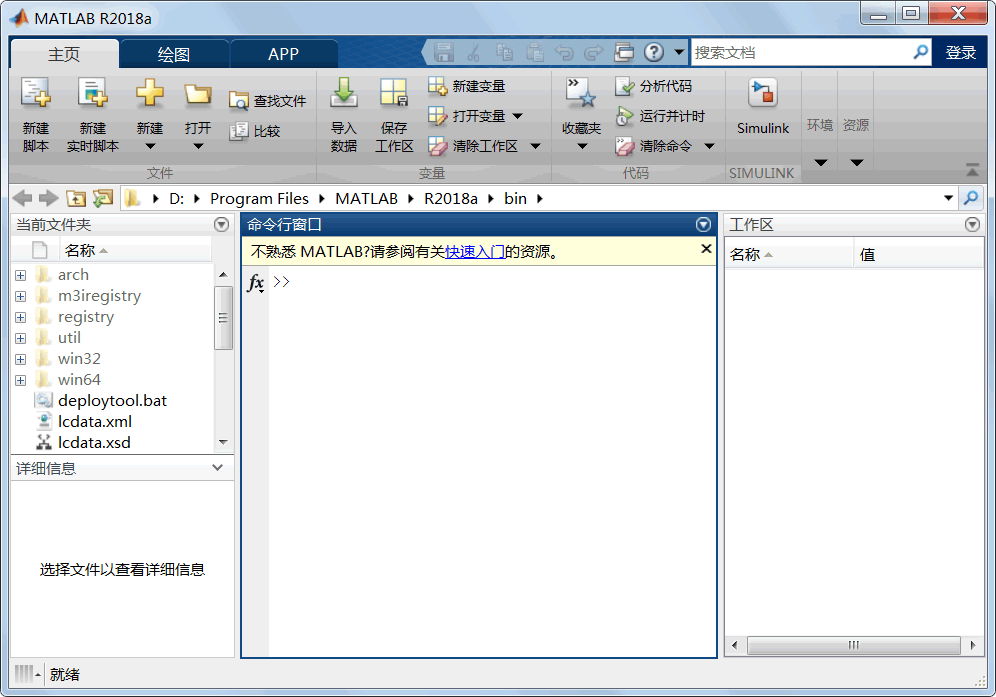## MATLAB简介

• 内容
• 评论
• 相关

MATLAB 是一款以数学计算为主的高级编程软件，提供了各种强大的数组运算功能用于对各种数据集合进行处理。

MATLAB 译为“矩阵实验室”，最初用来提供通往 LINPACK 和 EISPACK 矩阵的软件包接口。后来，它渐渐发展成为通用的科技计算图形交互系统和程序语言。

## MATLAB 简介

MATLAB 的基本数据单位是矩阵，它的指令表达与数学工程中常用的习惯形式十分相似，例如：

• 矩阵方程`Ax=b`在 MATLAB 中被写成`A*x=b`
• 而若要通过 A、b 求 x，那么只要写`x=b\A`即可完全不需要对矩阵的乘法和求逆进行编程。

MATLAB 发展到现在已经成为一个系列产品：MATLAB 主程序包和各种可选的 toolbox 工具包。主包中有数百个核心内部函数。迄今所有的四十几个工具包又可分为两类—功能性工具包和学科性工具包。

MATLAB 已经经过用户的多年考验。在欧美发达国家，MATLAB 已经成为应用线性代数、自动控制理论、数理统计、数字信号处理、时间序列分析、动态系统仿真等高级课程的基本教学工具，成为攻读学位的大学生、硕士生、博士生必须掌握的基本技能。在设计研究单位和工业部门，MATLAB 被广泛用于研究和解决各种具体工程问题。

MATLAB 的强大功能从本质上讲分为以下三类：

• 内部函数。
• 系统附带各种工具包中的M文件所提供的大量函数。
• 用户自己增加的函数。

MATLAB 提供的通用数理类函数包括如下：

• 基本数学函数。
• 特殊函数。
• 基本矩阵函数。
• 特殊矩阵函数。
• 矩阵分解和分析函数。
• 数据分析函数。
• 微分方程求解。
• 多项式函数。
• 非线性方程及其优化函数。
• 数值积分函数。
• 信号处理函数。

## MATLAB语言平台

MATLAB 支持许多操作系统，提供了大量的平台独立措施。在本文发布时，Windows XP、Windows 7、Windows 8 、Windows 10 和许多版本的 UNIX 系统都支持它。0条评论